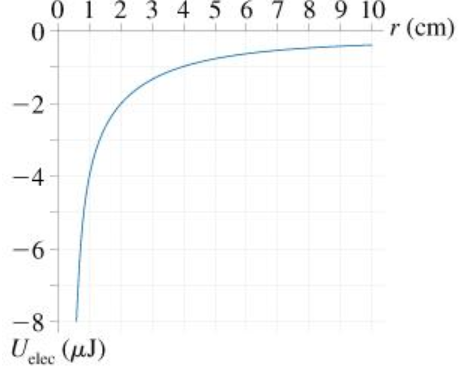# Problem: The graph in the figure shows the electric potential energy as a function of separation for two point charges.If one charge is +0.44 nC, what is the other charge?q=_____ nC

###### FREE Expert Solution

Potential energy:

$\overline{){\mathbf{U}}{\mathbf{=}}\frac{\mathbf{k}{\mathbf{q}}_{\mathbf{1}}{\mathbf{q}}_{\mathbf{2}}}{\mathbf{r}}}$

At the location, 1 cm, the potential energy of the two charges is - 4 μJ

97% (119 ratings)###### Problem Details

The graph in the figure shows the electric potential energy as a function of separation for two point charges.

If one charge is +0.44 nC, what is the other charge?

q=_____ nC